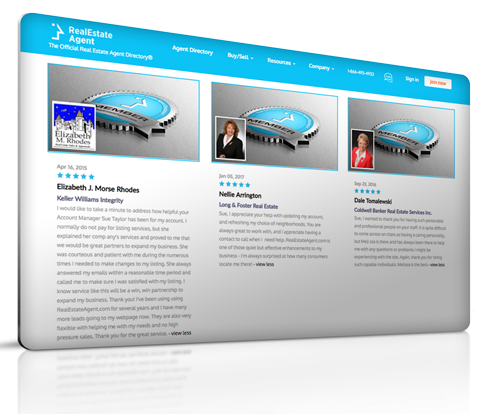# Cost-plus Contract

## Definition of "Cost-plus contract"

SUN REALTY
Estate Agents LLC, Real Estate Agent
.

Agreement in which the contract price to build something is equal to the total costs incurred plus a predetermined profit. The profit may be based on a percentage of cost (e.g., 20% of cost) or a flat profit figure (e.g., \$60,000). This type of contract is not good for the buyer because the contractor may intentionally overstate the construction costs to obtain a higher profit figure when profit is determined based on a percentage of total costs. If the construction costs are \$100,000 and the percentage of profit is 25%, the total contract price will be \$125,000.

#### Have a question or comment?We're here to help.